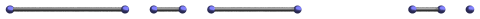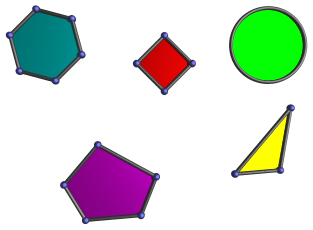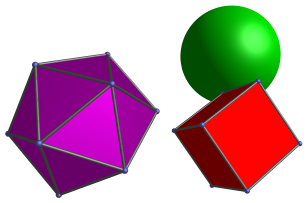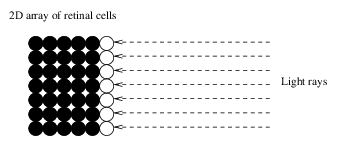# 4D Visualization

## Dimensional Analogy

A very useful tool in exploring 4D, or higher dimensions in general, is dimensional analogy. Dimensional analogy is the process of examining how a particular geometric feature in a lower dimension relates to an equivalent geometric feature in our dimension, and then applying the same principle to relate our dimension to a higher one.

Let's examine a few examples to see how this works.

### Boundaries of Objects

Let's begin with the very basics. Let's start in a 1D world. The 1D world is like a piece of string. There is only one axis along which one may move along this string, the X-axis. The only dimension any object can have is length, because there aren't any other dimensions to accomodate width or height. So the only possible objects in 1D are points, which are 0D, and lines, which are 1D.In order to completely specify a line segment, it is enough to specify its starting point and its ending point. In other words, the boundary of an object in 1D consists of points, which are 0D.

Now, let's move to the next higher dimension. The 2D world is a plane, like the surface of a piece of paper, but extending indefinitely in width and length. The 2D world is much more interesting than the 1D world, because a much larger variety of objects are possible. For example, we can have polygons and circles, in addition to points and lines:What is the boundary of a polygon? A polygon is bounded by line segments, which are 1D. A circle also has a 1D boundary: even though it is a curved boundary, it is fully specified by a single parameter: angle. So 2D objects are bounded by 1D lines and curves.

Now let's move on to the 3D world. Objects in the 3D world are bounded not by lines or curves, but by 2D surfaces. For example, a cube is bounded by 6 squares, and a ball is bounded by a spherical surface. The spherical surface is 2D, because any point on the sphere is fully specified by only two parameters: longitude and latitude.We can see a pattern emerge here. Objects in 1D are bounded by 0D points; objects in 2D are bounded by 1D lines (or curves); and objects in 3D are bounded by 2D surfaces. In other words, points in 1D are analogous to lines and curves in 2D: they form the boundaries of objects in the respective dimensions. Similarly, bounding lines and curves in 2D are analogous to surfaces in 3D. So, by applying dimensional analogy, we see that in N dimensions, objects are bounded by (N-1)-dimensional boundaries.

This leads us to conclude that in 4D, objects are bounded not by points, lines, nor even surfaces, but volumes. It would be rather difficult to realize this without applying dimensional analogy. For example, as we shall see later, a 4D cube is bounded by 8 cubes. We call these bounding volumes the cells of the 4D cube.

### Vision

Another application of dimensional analogy that has been mentioned before is the dimension of the retina in the eye of an N-dimensional creature. We are 3D beings, yet our eyes only see in 2D because our retina is only a 2D array of light-sensitive cells.

Why are our retinas only 2D? Surely it would be much better for us to have a 3D retina, so that we can see every part of our 3D world simultaneously?

The reason is that in order for us to see something, light must have an unobstructed path from that thing to the cells in our retina. A 2D retina works, because there is a 3rd dimension in which the light can travel unobstructed from the object onto the retina. However, if our retina were 3D, we would not see anything more, because light must pass the cells on the outer surface in order to reach the inner cells, so that what the inner cells see has already been seen by the outer cells. Since we are confined to 3D, there is no additional dimension in which light may travel to reach these inner cells by an independent path, which might have given us additional visual information.This fact leads us to conclude, by dimensional analogy, that a 2D creature must only have a 1D retina. As the diagram above shows, when a creature is confined to 2D, there is no unobstructed path for light to travel from a 2D object to an inner cell in a 2D retina. Any light that reaches an inner cell has already passed an outer cell, so having a 2D retina would not help the creature to see more.

We can also conclude by dimensional analogy that in 4D, it is possible to have a 3D retina, because there is now an extra dimension in which light can travel unobstructed from the object being seen to any point on the retina.

We shall make much use of dimensional analogy to understand 4D in the subsequent discussions.

Last updated 06 Feb 2018.